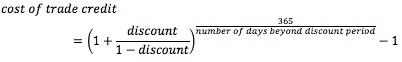### Seeing is believing!

Before you order, simply sign up for a free user account and in seconds you'll be experiencing the best in CFA exam preparation.

##### Subject 4. Evaluating Accounts Receivable, Inventory and Accounts Payable Management
Accounts Receivable

The most popular measures to evaluate receivables are receivable turnover and number of days of receivables.

Example

Build It Right, Inc. sells 5,500 curio cabinets a year at a price of \$2,000 each. The credit terms of the sale are 2/10, net 45. Eighty percent of the firm's customers take the discount. What is the amount of the firm's accounts receivable?

If 80% of the customers pay in 10 days, then the other 20% must pay in 45 days.
How much does the firm sell each year? 5,500 x \$2,000 = \$11,000,000
The average collection period: [0.80 x 10] + [(1 - 0.80) x 45] = 8 + 9 = 17 days.
The accounts receivable turnover: 365 / 17 = 21.470588.
The average receivables balance: \$11,000,000 / 21.470588 = \$512,328.77.

Inventory

Managing inventory is a juggling act. Excessive stocks can place a heavy burden on the cash resources of a business. Insufficient stocks can result in lost sales, delays for customers, etc. The goal of inventory management is to identify the level of inventory which allows for uninterrupted production but reduces the investment in raw materials - and minimizes reordering costs - and hence increases cash flow.

Just-In-Time (JIT) is an inventory strategy implemented to improve a business's return on investment by reducing in-process inventory and its associated costs. Economic order quantity (also known as the EOQ Model) is a model that defines the optimal quantity to order that minimizes total variable costs required to order and hold inventory.

To evaluate inventory management analysts compute the inventory turnover ratio and the number of days of inventory. These measures are covered in Study Session 7.

Accounts Payable

Two countering forces should be considered when managing accounts payable:

• Paying too early is costly unless the company can take advantage of discounts.
• Postponing payment beyond the end of the net (credit) period is known as "stretching accounts payable" or "leaning on the trade." Possible costs are:

• Cost of the cash discount (if any) forgone.
• Late payment penalties or interest.
• Deterioration in credit rating.

Trade discounts should be evaluated by computing the implicit rate of return:Example

Today, June 10, you purchased \$5,000 worth of materials from one of your suppliers. The terms of the sale are 3/15, net 45.

• Discounted price: \$5,000 x (1 - 0.03) = \$4,850
• Last day to receive discount: June 10 + 15 days = June 25
• Days credit: 45 - 15 = 30
• Implicit interest: 0.03 x \$5,000 = \$150
• Cost of credit (effective annual rate): (1 + 0.03/0.97)365/30 - 1 = 44.86%

Analysts often use the number of days of payables and payables turnover to evaluate accounts payable management.

• Payables turnover = Total purchases / Accounts payables
• Number of days of payables = # of days in period / Payables turnover = Accounts payable / Average day's purchase

Learning Outcome Statements

f. evaluate a company's management of accounts receivable, inventory, and accounts payable over time and compared to peer companies;

CFA® 2022 Level II Curriculum, , Volume 4, Reading 35

User Comment
davidvcohen In the first example with account receivables, shouldn't we consider the 2% discount to calculate the receivables turnover? That way, the firm would sell less than the 11.000 a year.
javasun it's already considered in the probability calculations.
8thlegend What is the 44.86% representing? It's going to cost 44.86% more if you dont pay for the discount or what?
shiva5555 What does 2/10 net 45 mean?
teje 2/10 net 45 means customer is entitled to a 2% discount if paid within 10 days, or else the amount is due in 45 days (with no discount)
thekobe 8thlegend, if you taje a look at quantitative methods you will see that in order to convert a short term rate into an effective you do some similar procedures HPY=(discount/face-discount)
johntan1979 Q* = (2DS/H)^1/2
johntan1979 And to answer 8thlegend's question, if the cost of credit (44.86%) exceeds the short-term borrowing rate, the firm should take the discount even if it means the firm need to borrow short-term to do so.
SalimBouch javasun, what do you mean by probability calculations here? I don't see where the 2% discount was taken into account in the first example.. thanks in advance for your help
dlackie 80% of customers paid in 10 days. We are looking to calculate average AR during the period, not cash received. So the 2% doesn't matter in calculating average collection period.
zriddle the 44.86% is the annual rate of return that the discount represents.
mcbreatz Just to cement this concept in my brain. When it comes to the 44.86% the short term rate is actually what? And then we would compare to short term rate for borrowing? Is the short term implied rate 3% in this example?